Objectives: :

• - Express large and small numbers in scientific notation.
• - Use your GDC (Graphic Display Calculator) to find numbers in scientific notation.
##### 1.

Your content goes here. Edit or remove this text inline or in the module Content settings. You can also style every aspect of this content in the module Design settings and even apply custom CSS to this text in the module Advanced settings.

##### 0. Decimal places & Significant figures

Consider the number:

## 123.4567

There are two ways to round up the number by using fewer digits:
1. In a specific number of decimal places (d.p.)

in 1 d.p. 123.5
in 2 d.p. 123.46
in 3 d.p. 123.457

2. In a specific number of significant figures (s.f.). For the position of cutting we start counting from the first non-zero digit:

in 4 s.f. 123.5in 5 s.f. 123.46
Inchangé : in 6 s.f. 123.457

But also

in 2 s.f. 120
Inchangé : in 1 s.f. 100

Important remark: In the final IB exams the requirement is to give Inchangé : the answers either in exact form or in 3 s.f.

For example:

$$2\pi$$6.28

 Exact form in 3 s.f. $$\sqrt{2}$$ 1.41 12348 12300

### METHOD:

The method is the same as with decimal places except that you start counting from the Inchangé : very beginning of the number (instead of just after the decimal point):

- If 5 or above then round the last number up.

- If 4 or below the last number stays the same

Examples:

a. Round 15.748 to 3 significant figures.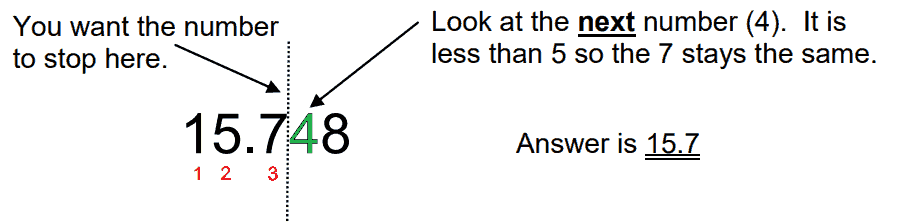b. Round 64.3463 to 2 significant figures.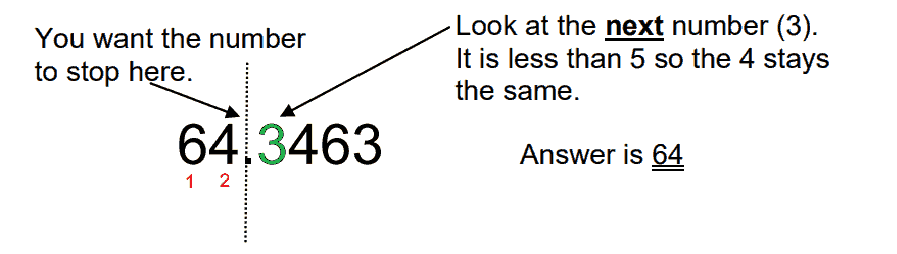c. Round 128.35 to 2 significant figures.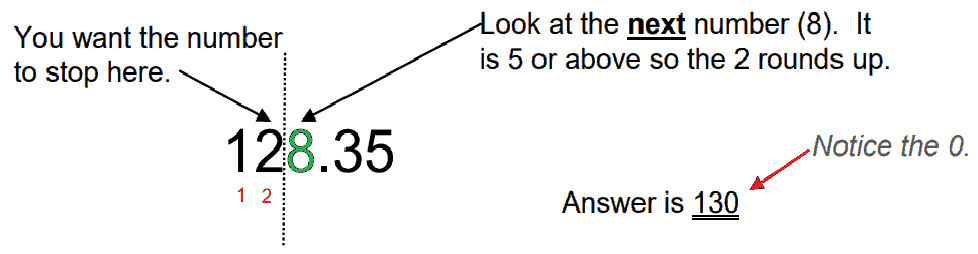Exercise

##### 1. Powers of 10
Find each of these values as a power of 10.

Example : 1 000 = 103

Find each of these values as a power of 10.

Example : 0.001 = 10-3

# For a positive integer n :

• $$10^{\color{red}{n}}$$ is written with a 1 followed by n zeros.
• $$10^{\color{green}{-n}}$$ is written with a 1 preceded by n zeros.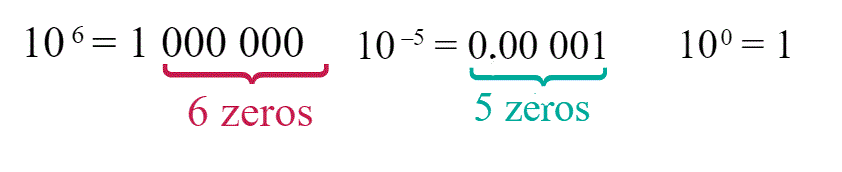Exercise :

##### 2. Scientific notation

How can we use our findings so far to express numbers as multiple of powers of ten .

For example $$8, 000$$ :

$$8, 000 = 8 \times 1,\color{green}{000}$$ and we know$$1, \color{green}{000} = 10^{\color{green}{3}}$$

so, $$8, \color{green}{000} = 8\times10^{\color{green}{3}}$$

what about $$60 ,000 ,000$$ ?

$$6\color{green}{0, 000 ,000 }= 6\times 1\color{green}{0 ,000 ,000}$$

$$6\color{green}{0, 000, 000}= 6\times 10^{\color{green}{7}}$$

and $$0.005$$?

$$\color{red}{ 0.00}5=5\times \color{red}{ 0.00}1$$

$$\color{red}{ 0.00}5=5\times 10^{\color{red}{ -3}}$$

# Scientific Notation

## $$a \times 10^k$$ where $$1 \leq a<10$$ and $$k\in \mathbb{Z}$$

### METHOD:

#### Write 427 000 000 000 in scientific notation:

1. Move the decimal place to the left to create a new number from 1 up to 10. Inchangé :
• The decimal point is at the end of the number:
• 427 000 000 000. So, $$a=4\color{red}{.}27$$
2. Determine the exponent, which is the number of times you moved the decimal point.
• In this example, you moved the decimal 11 times; also, because you moved the decimal to the left, the exponent is positive. Therefore, k = 11, and so you get $$10^{11}$$
3. Put the number in the correct form for scientific notation
• $$a \times 10^{k}$$
• $$4.27\times10^{11}$$

#### Write 0.00 000 037 in scientific notation.

1. Move the decimal place to the right to create a new number from 1 up to 10.
• So $$a=3\color{green}{.}7$$
2. Determine the exponent, which is the number of times you moved the decimal point.
• In this example, you moved the decimal 7 times; also, because you moved the decimal to the right, the exponent is negative. Therefore, $$a = –7$$, and so you get $$10^{-7}$$
3. Put the number in the correct form for scientific notation
• $$a \times 10^k$$
• $$3.7\times10^{-7}$$

### 1.325E79 means $$1.325\times 10^{79}$$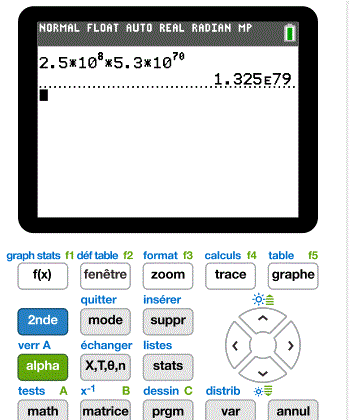Exercise :

### $$3.2045×10^{-5}≅3.20×10^{-5}$$

##### Teachers Ressources

Quizizz as a warm up or at the end of your course. This resource can be used by the students themselves by clicking on the "practice" button:

 - Significant figures